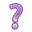## 12.3 Predicted Class (outcome)

To classify individual $$i$$ as being depressed or not, we draw a binary value ($$x_{i} = 0$$ or $$1$$), with probability $$p_{i}$$ by using the rbinom function, with a size=1.

set.seed(12345) #reminder: change the combo on my luggage
plot.mpp <- data.frame(pred.prob = phat.depr,
pred.class = rbinom(n = length(phat.depr),
size = 1,
p = phat.depr),
truth = dep_sex_model$y) head(plot.mpp) ## pred.prob pred.class truth ## 1 0.21108906 0 0 ## 2 0.08014012 0 0 ## 3 0.15266203 0 0 ## 4 0.24527840 1 0 ## 5 0.15208679 0 0 ## 6 0.17056409 0 0 Applying class labels and creating a cross table of predicted vs truth: plot.mpp <- plot.mpp %>% mutate(pred.class = factor(pred.class, labels=c("Not Depressed", "Depressed")), truth = factor(truth, labels=c("Not Depressed", "Depressed"))) table(plot.mpp$pred.class, plot.mpp\$truth)
##
##                 Not Depressed Depressed
##   Not Depressed           195        35
##   Depressed                49        15

The model correctly identified 195 individuals as not depressed and 15 as depressed. The model got it wrong 49 + 35 times.

The accuracy of the model is calculated as the fraction of times the model prediction matches the observed category:

(195+15)/(195+35+49+15)
##  0.7142857

This model has a 71.4% accuracy.Is this good? What if death were the event?